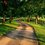# [Set Theory] Proving Cantor's Theorem by Contradicting Surjectivity on Power Sets

Let $$S$$ be a set, and let $$P(S )$$ be the power set of $$S$$, which is the set of subsets of $$S$$.

Suppose that $f: S \rightarrow P(S )$ surjects. We define $T = \{ x\in S: x \not\in f(x) \}.$ so that $f(x)$ is an element of $P(S)$, hence a subset of $S$, and $x$ is an element of $S$.

By assumption $f$ surjects, so there exists $t \in S$ such that $f (t) = T$.

First, let $t \in T$. Then $t \in \{ x \in S: x \not\in f(x) \},$

so $t \in S$ such that $t \not\in f(t)$. But since $t \in T= f(t)$, this is a contradiction because $t \in T = f(t)$, but $t \not\in f(t)$.

Now let $t \not\in T$. Then since $t$ is not in $T$, $t$ cannot be an element of $S$ such that $t \not\in f(x)$. So if $t \in S$, then $t$ must also be a member of $f(t)$. But supposing that $f$ surjects, there is a $t \in S$ such that $f(t) = T$, which means that $t \not\in T = f(t)$, but $t \in f(t)$. This is a contradiction.

$\therefore$ We conclude from both cases that $f$ cannot surject, because there exists no $t \in S$ such that $f(t) = T$. In other words, $T \not= f(t)$ for all $t \in S$.

In this note, we have shown that no map from set $S$ to its power set $P(S )$ can surject, which proves that there exists no bijection from S to its power set. This fundamental result means that for any set S, the set of all subsets of $S$, $P(S)$,has a strictly greater cardinality than $S$. That

$|S| < |P(S)|$ or $Card(S) < Card(P(S)).$Note by Bright Glow
3 years, 1 month ago

This discussion board is a place to discuss our Daily Challenges and the math and science related to those challenges. Explanations are more than just a solution — they should explain the steps and thinking strategies that you used to obtain the solution. Comments should further the discussion of math and science.

When posting on Brilliant:

• Use the emojis to react to an explanation, whether you're congratulating a job well done , or just really confused .
• Ask specific questions about the challenge or the steps in somebody's explanation. Well-posed questions can add a lot to the discussion, but posting "I don't understand!" doesn't help anyone.
• Try to contribute something new to the discussion, whether it is an extension, generalization or other idea related to the challenge.
• Stay on topic — we're all here to learn more about math and science, not to hear about your favorite get-rich-quick scheme or current world events.

MarkdownAppears as
*italics* or _italics_ italics
**bold** or __bold__ bold
- bulleted- list
• bulleted
• list
1. numbered2. list
1. numbered
2. list
Note: you must add a full line of space before and after lists for them to show up correctly
paragraph 1paragraph 2

paragraph 1

paragraph 2

[example link](https://brilliant.org)example link
> This is a quote
This is a quote
    # I indented these lines
# 4 spaces, and now they show
# up as a code block.

print "hello world"
# I indented these lines
# 4 spaces, and now they show
# up as a code block.

print "hello world"
MathAppears as
Remember to wrap math in $$ ... $$ or $ ... $ to ensure proper formatting.
2 \times 3 $2 \times 3$
2^{34} $2^{34}$
a_{i-1} $a_{i-1}$
\frac{2}{3} $\frac{2}{3}$
\sqrt{2} $\sqrt{2}$
\sum_{i=1}^3 $\sum_{i=1}^3$
\sin \theta $\sin \theta$
\boxed{123} $\boxed{123}$

## Comments

Sort by:

Top Newest

You need to work in something like $\text{ZF}$ to guarantee the diagonal construction, and as mentioned below show that there be an injection $S\longrightarrow\mathcal{P}(S)$. Your proof is otherwise standard and fine. I would write it more compactly as follows:

Claim. There is no surjection $f:S\longrightarrow\mathcal{P}(S)$. Proof. Let $f:S\longrightarrow P(S)$. To show: $f$ is not surjective. By the replacement axiom there exists a set $\Delta:=\{x\in S\mid x\notin f(x)\}\in\mathcal{P}(S)$. It suffices to show that $\Delta\notin\text{ran}(f)$. Suppose this not be the case, then there exists $\delta\in S$ such that $f(\delta)=\Delta$. It holds that $\delta\in f(\delta)$ $\overset{f(\delta)=\Delta}{\Longleftrightarrow}$ $\delta\in\Delta$ $\overset{\text{defn. \\Delta\}}{\Longleftrightarrow}$ $\delta\notin f(\delta)$. This is a contradiction. Hence $\Delta\notin\text{ran}(f)$. $\blacksquare$

Note that without suitable axioms, one cannot carry through with Cantor’s diagonal argument. In under Quine’s $\text{NF}$ set theory this proof breaks down right at this point, and not only this, there exists sets such that $|\mathcal{P}(X)|=|X|$.

- 3 years ago

Log in to reply

You may also want to show that $f$ is injective. Otherwise, it is not obvious that $|S| \leq |P(S)|$. (The surjective argument only shows that the cardinalities are not equal.)

- 3 years, 1 month ago

Log in to reply

Thanks for pointing that out! Following your comment, constructing $g: S \rightarrow P(S)$ so that every element of $S$ is mapped to a singleton set, i.e. $x \rightarrow \{x\}$ will do.

- 3 years, 1 month ago

Log in to reply

×

Problem Loading...

Note Loading...

Set Loading...